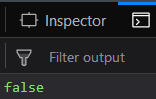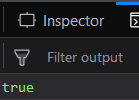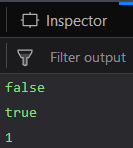# Underscore.js | _.isNull()

_.isNull() function:

• It is used to find whether the value of the object is null.
• If object has null value then the output will be true otherwise false.
• We can even perform addition, subtraction etc operations in this function.

Syntax:

`_.isNull(object)`

Parameters:
It takes only one argument which is the object that needs to be tested.
Return value:
It returns true if the object has null value otherwise it returns false.

Examples:

1. Passing a number in the _.isNull() function:
The _.isNull() function takes the parameter passed to it and then checks whether the object has null value or not. In this case since the value is a defined number “10”, so the output is not null. Therefore, the output will be false.

 `` `<``html``> ` `   `  `<``head``> ` `    ``<``script` `src` `=  ` `"https://cdnjs.cloudflare.com/ajax/libs/underscore.js/1.9.1/underscore-min.js"` `> ` `    `` ` ` ` `   `  `<``body``> ` `    ``<``script` `type``=``"text/javascript"``> ` `        ``var a=10; ` `        ``console.log(_.isNull(10)); ` `    `` ` ` ` `   `  ` `

Output:2. Passing “null” to the _.isNull() function:
Since here we have passed “null” so we need not check for the object. We know that the value passed to the _.isNull() function itself has value “null”. Therefore, the output will be true.

 `` `<``html``> ` `   `  `<``head``> ` `    ``<``script` `src` `=  ` `"https://cdnjs.cloudflare.com/ajax/libs/underscore.js/1.9.1/underscore-min.js"` `> ` `    `` ` ` ` `   `  `<``body``> ` `    ``<``script` `type``=``"text/javascript"``> ` `        ``console.log(_.isNull(null)); ` `    `` ` ` ` `   `  ` `

Output:3. Passing undefined” to the _.isNull() function:
The _.isNull() function takes the parameter passed to it which here is “undefined”. We know that if anything is undefined, then it’s value will be null. And hence, the answer is true.

 `` `<``html``> ` `   `  `<``head``> ` `    ``<``script` `src` `=  ` `"https://cdnjs.cloudflare.com/ajax/libs/underscore.js/1.9.1/underscore-min.js"` `> ` `    `` ` ` ` `   `  `<``body``> ` `    ``<``script` `type``=``"text/javascript"``> ` `        ``console.log(_.isNull(undefined)); ` `    `` ` ` ` `   `  ` `

Output:4. Performing operations on the output of _.isNull() function:
In this first we have directly stored the output of both the above 2 examples (2, 3) in variables a and b, and then we have done an addition operation in both the results. Finally, stored it in the third variable. Since the output of _.isNull() is false when we passed and true when we passed null, therefore false is stored in ‘a’ variable and true is stored in ‘b’ variabe. Now if we perform addition (+) operation on both the ‘a’, ‘b’ variables then we will have true as ‘b’ is true. Hence ‘c’ variable will become 1.

## Recommended: Please try your approach on {IDE} first, before moving on to the solution.

 `` `<``html``> ` `   `  `<``head``> ` `    ``<``script` `src` `=  ` `"https://cdnjs.cloudflare.com/ajax/libs/underscore.js/1.9.1/underscore-min.js"` `> ` `    `` ` ` ` `   `  `<``body``> ` `    ``<``script` `type``=``"text/javascript"``> ` `        ``var a = _.isNull(undefined); ` `        ``var b = _.isNull(null); ` `        ``var c = a + b; ` `        ``console.log(a); ` `        ``console.log(b); ` `        ``console.log(c); ` `    `` ` ` ` `   `  ` `

Output:NOTE: These commands will not work in Google console or in firefox as for these additional files need to be added which they didn’t have added.

 `` `<``script` `type``=``"text/javascript"` `src` `= ` `"https://cdnjs.cloudflare.com/ajax/libs/underscore.js/1.9.1/underscore-min.js"``> ` ` `My Personal Notes arrow_drop_upCheck out this Author's contributed articles.

If you like GeeksforGeeks and would like to contribute, you can also write an article using contribute.geeksforgeeks.org or mail your article to contribute@geeksforgeeks.org. See your article appearing on the GeeksforGeeks main page and help other Geeks.

Please Improve this article if you find anything incorrect by clicking on the "Improve Article" button below.